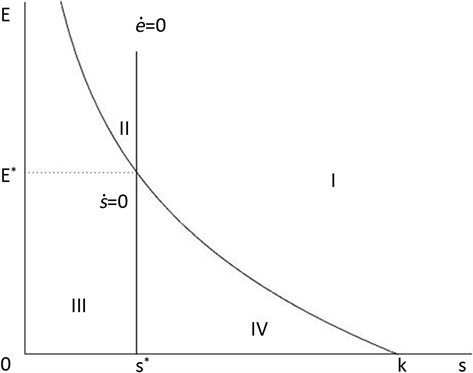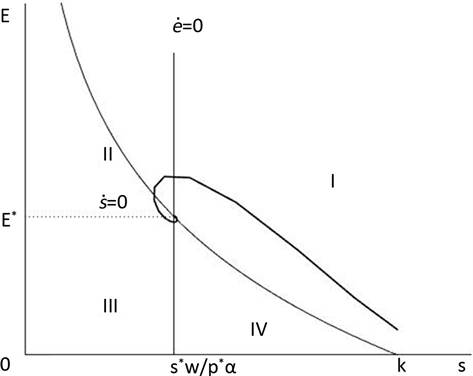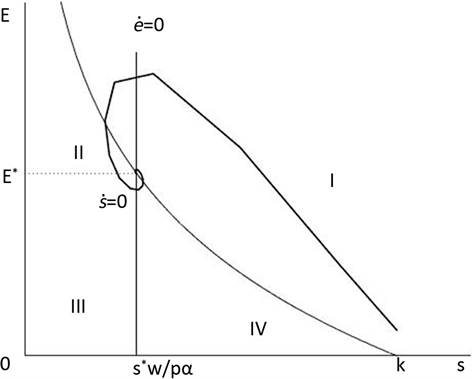﻿ 动态价格下Gompertz系统的捕捞问题

# 动态价格下Gompertz系统的捕捞问题Harvesting in Gompertz System with Dynamic Price

Abstract: In this paper, a harvesting model with price changed by market inside control is established for open fishery. The only equilibrium is proved to be positive and stable. We analyze the dynamics of fishery and draw a conclusion that the population of biological species in equilibrium decreasing and fishing effort increasing when the constant price is greater than the equilibrium price. At last, we provide government departments with guidance in control price.

1. 引言

$\left\{\begin{array}{l}\frac{\text{d}s}{\text{d}t}=F\left(s\right)-Es,\\ \frac{\text{d}E}{\text{d}t}=v\left(pEs-wE\right),\end{array}$ (1)

$p\left(S\right)=\frac{n}{m+S},$

$\underset{S\to 0}{\mathrm{lim}}p\left(S\right)=\frac{n}{m}={p}_{\mathrm{max}},\text{\hspace{0.17em}}\underset{S\to \infty }{\mathrm{lim}}p\left(S\right)=0,$

$\left\{\begin{array}{l}\frac{\text{d}s}{\text{d}t}=F\left(s\right)-\alpha Es,\\ \frac{\text{d}E}{\text{d}t}=v\alpha Es\left(\frac{n}{m+\alpha Es}-\frac{w}{\alpha s}\right),\end{array}$ (2)

$\frac{\text{d}p}{\text{d}t}=\beta \left({q}^{d}-{q}^{s}\right),\beta >0.$ (3)

$\frac{\text{d}p}{\text{d}t}=\beta \left(a-c\right)+\beta \left(b-d\right)p.$ (4)

$\left\{\begin{array}{l}\frac{\text{d}s}{\text{d}t}=rs\mathrm{ln}\left(k/s\right)-\alpha Es,\\ \frac{\text{d}E}{\text{d}t}=v\pi =v\left(p\alpha Es-wE\right),\\ \frac{\text{d}p}{\text{d}t}=\beta \left(a-c\right)+\beta \left(b-d\right)p,\end{array}$ (5)

2. 平衡点及其稳定性分析

$\left\{\begin{array}{l}rs\mathrm{ln}\left(k/s\right)-\alpha Es=0,\\ p\alpha Es-wE=0,\\ \beta \left(a-c\right)+\beta \left(b-d\right)=0,\end{array}$

${E}_{*}=\left({s}^{*},{E}^{*},{p}^{*}\right).$

${s}^{*}=\frac{w}{{p}^{*}\alpha }$${E}^{*}=\frac{r}{\alpha }\mathrm{ln}\left(k/{s}^{*}\right)$${p}^{*}=\frac{a-c}{d-b}$

$J=\left(\begin{array}{ccc}r\mathrm{ln}\left(k/s\right)-r-\alpha E& -\alpha s& 0\\ vp\alpha E& v\left(p\alpha s-w\right)& v\alpha Es\\ 0& 0& \beta \left(b-d\right)\end{array}\right)$

${a}_{0}{\lambda }^{3}+{a}_{1}{\lambda }^{2}+{a}_{2}\lambda +{a}_{3}=0,$

${a}_{0}=1,{a}_{1}=r-\beta \left(b-d\right)$

${a}_{2}=\left[vwr\mathrm{ln}\left(k/{s}^{*}\right)-r\beta \left(b-d\right)\right]$

${a}_{3}=-\beta \left(b-d\right)vwr\mathrm{ln}\left(k/{s}^{*}\right)$

$\begin{array}{c}{\Delta }_{2}=\left[r-\beta \left(b-d\right)\right]\left[vwr\mathrm{ln}\left(k/{s}^{*}\right)-r\beta \left(b-d\right)\right]+\beta \left(b-d\right)vwr\mathrm{ln}\left(k/{s}^{*}\right)\\ =r\left[vwr\mathrm{ln}\left(k/{s}^{*}\right)-r\beta \left(b-d\right)\right]+r{\beta }^{2}{\left(b-d\right)}^{2}\end{array}$

3. 开放渔业的动力学行为

4. 小结Table 1. Increasing or decreasing of population and effort in four quadrantsFigure 1. Equilibrium of population and equilibrium of effort divide the area into four quadrantsFigure 2. Derived from model (5), the trajectory of the system over time in equilibrium priceFigure 3. Derived from model (1), the trajectory of the system over time in constant price

 Clark, C.W. (1990) Mathematical Bioeconomics. 2nd Edition. John Whiley, New York.

 Shone, R. (2002) Economic Dynamics Phase Diagrams and Their Economic Application. 2nd Edition. Cambridge University Press, New York.

 范猛, 王克. 价格随供求变化的捕捞问题[J]. 生物数学学报, 2001, 16(4): 411-415.

 高海音, 敬石心, 朱天晓. 价格成本变化的Gompertz系统的捕捞问题[J]. 长春大学学报, 1999, 9(3): 13-14.

 吴秀兰, 朱宏. 关于一类价格随供求变化的捕捞模型的分析[J]. 吉林师范大学学报(自然科学版), 2006(3): 73-74.

 陈静. 价格随供求变化的捕食者–食饵生态经济系统的Hopf分支与最优捕捞[D]: [硕士学位论文]. 武汉: 湖北师范学院, 2014.

 刚毅, 雒志学. 价格随供求变化经济捕捞模型的定性分析[J]. 河南科技大学学报: 自然科学版, 2007, 28(2): 102-104.

 魏凤英, 王克. 价格成本变化的具有smith增长和崔一L增长的生物种群的经济捕捞模型[J]. 生物数学学报, 2004, 19(3): 328-336.

 孙承毅, 张树文. 变价格下的单向捕捞模型[J]. 鞍山师范学院学报, 1999, 1(4): 69-73.

Top